## Acceleration

Let a particle travel a distance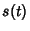as a function of time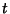(here,can be thought of as the Arc Length of the curve traced out by the particle). The Speed (the Scalar Norm of the Vector Velocity) is then given by(1)

The acceleration is defined as the time Derivative of the Velocity, so the Scalar acceleration is given by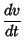(2)(3)(4)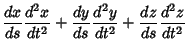(5)(6)

The Vector acceleration is given by(7)

where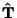is the Unit Tangent Vector,the Curvature,the Arc Length, andthe Unit Normal Vector.

Let a particle move along a straight Line so that the positions at times,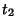, andare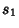,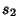, and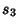, respectively. Then the particle is uniformly accelerated with accelerationIff(8)

is a constant (Klamkin 1995, 1996).

Consider the measurement of acceleration in a rotating reference frame. Apply the Rotation Operator(9)

twice to the Radius Vector r and suppress the body notation,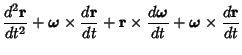(10)

Grouping terms and using the definitions of the Velocity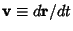and Angular Velocity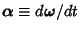give the expression(11)

Now, we can identify the expression as consisting of three terms(12)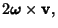(13)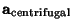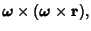(14)

a body'' acceleration, centrifugal acceleration,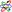and Coriolis acceleration.Using these definitions finally gives(15)

where the fourth term will vanish in a uniformly rotating frame of reference (i.e.,). The centrifugal accelerationis familiar to riders of merry-go-rounds, and the Coriolis accelerationis responsible for the motions of hurricanes on Earthand necessitates large trajectory corrections for intercontinental ballistic missiles.

Klamkin, M. S. Problem 1481.'' Math. Mag. 68, 307, 1995.
Klamkin, M. S. A Characteristic of Constant Acceleration.'' Solution to Problem 1481. Math. Mag. 69, 308, 1996.